# Chemistry The Central Science 11 th edition Theodore

• Slides: 50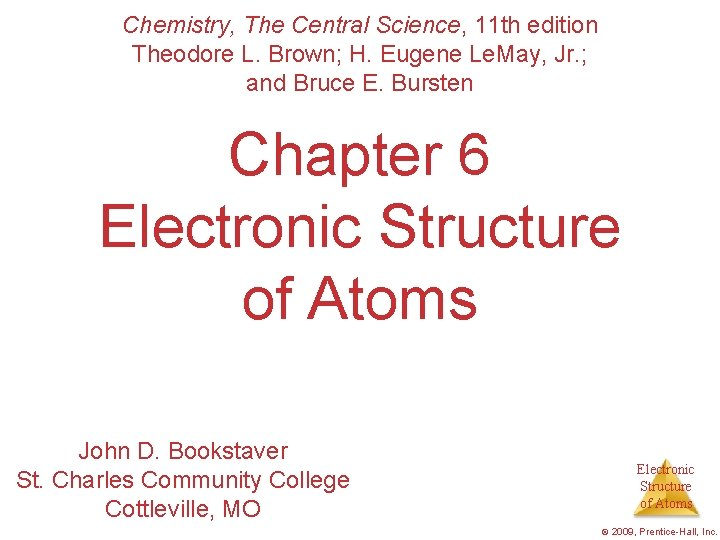Chemistry, The Central Science, 11 th edition Theodore L. Brown; H. Eugene Le. May, Jr. ; and Bruce E. Bursten Chapter 6 Electronic Structure of Atoms John D. Bookstaver St. Charles Community College Cottleville, MO Electronic Structure of Atoms © 2009, Prentice-Hall, Inc.Aim: How does light act as a wave? Do Now: All light travels through a vacuum at what speed? Electronic Structure of Atoms © 2009, Prentice-Hall, Inc.Waves • To understand the electronic structure of atoms, one must understand the nature of electromagnetic radiation. • The distance between corresponding points Electronic on adjacent waves is the wavelength ( ). Structure of Atoms © 2009, Prentice-Hall, Inc.Waves • The number of waves passing a given point per unit of time is the frequency ( ). • For waves traveling at the same velocity, the longer the wavelength, the smaller the frequency. Electronic Structure of Atoms © 2009, Prentice-Hall, Inc.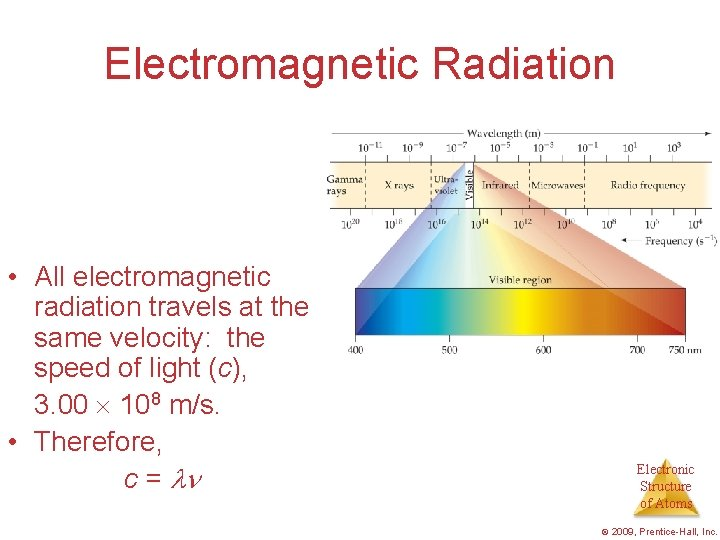Electromagnetic Radiation • All electromagnetic radiation travels at the same velocity: the speed of light (c), 3. 00 108 m/s. • Therefore, c = Electronic Structure of Atoms © 2009, Prentice-Hall, Inc.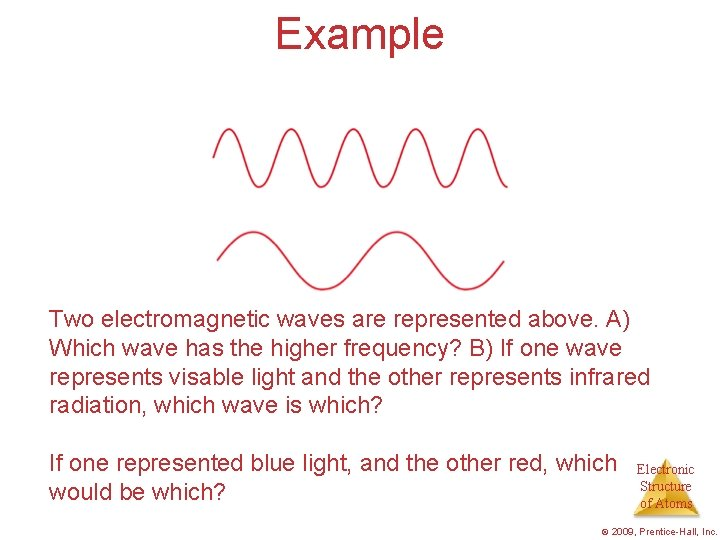Example Two electromagnetic waves are represented above. A) Which wave has the higher frequency? B) If one wave represents visable light and the other represents infrared radiation, which wave is which? If one represented blue light, and the other red, which would be which? Electronic Structure of Atoms © 2009, Prentice-Hall, Inc.Example What if wavelength is given in nm? What must we do with the speed of light c? (3. 00 x 108 m/s) Electronic Structure of Atoms © 2009, Prentice-Hall, Inc.Pg. 250 Ex. 6. 21 • A laser pointer used in a lecture hall emits light at 650 nm. What is the frequency of this radiation? Using Figure 6. 4 predict the color associated with this wavelength. Electronic Structure of Atoms © 2009, Prentice-Hall, Inc.The Nature of Energy • The wave nature of light does not explain how an object can glow when its temperature increases. • Max Planck explained it by assuming that energy comes in packets called quanta. Electronic Structure of Atoms © 2009, Prentice-Hall, Inc.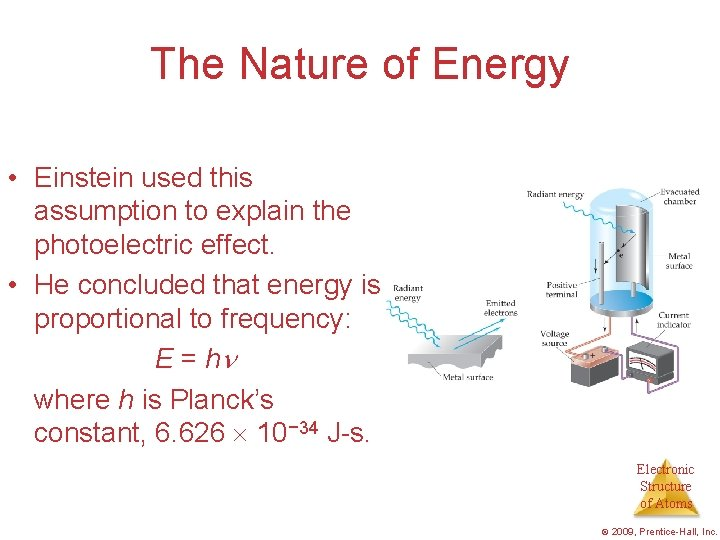The Nature of Energy • Einstein used this assumption to explain the photoelectric effect. • He concluded that energy is proportional to frequency: E = h where h is Planck’s constant, 6. 626 10− 34 J-s. Electronic Structure of Atoms © 2009, Prentice-Hall, Inc.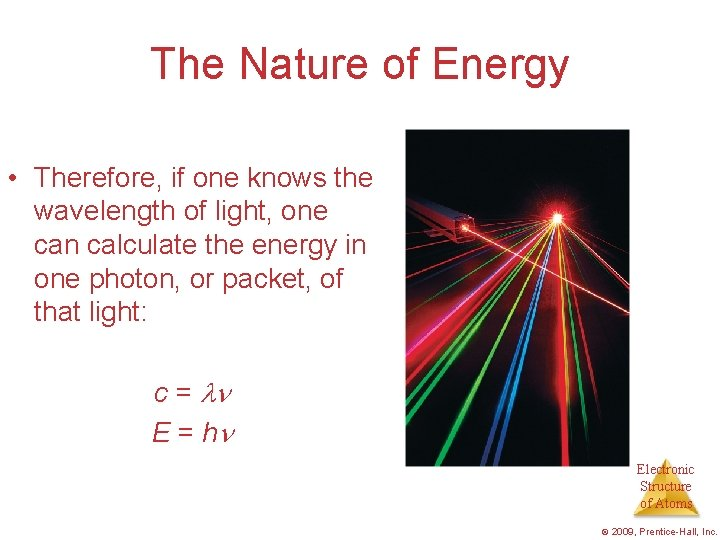The Nature of Energy • Therefore, if one knows the wavelength of light, one can calculate the energy in one photon, or packet, of that light: c = E = h Electronic Structure of Atoms © 2009, Prentice-Hall, Inc.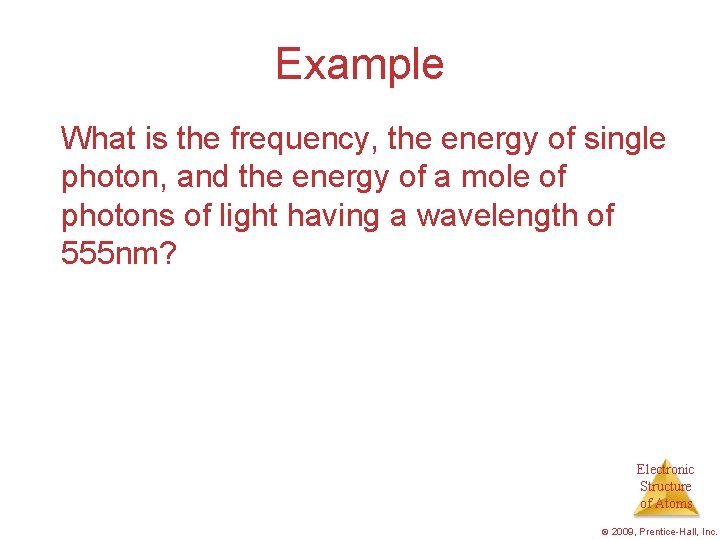Example What is the frequency, the energy of single photon, and the energy of a mole of photons of light having a wavelength of 555 nm? Electronic Structure of Atoms © 2009, Prentice-Hall, Inc.The Nature of Energy Another mystery in the early 20 th century involved the emission spectra observed from energy emitted by atoms and molecules. Electronic Structure of Atoms © 2009, Prentice-Hall, Inc.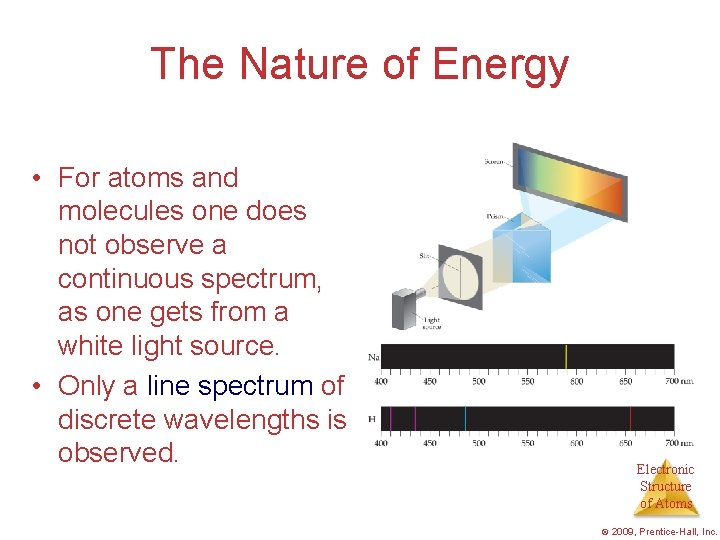The Nature of Energy • For atoms and molecules one does not observe a continuous spectrum, as one gets from a white light source. • Only a line spectrum of discrete wavelengths is observed. Electronic Structure of Atoms © 2009, Prentice-Hall, Inc.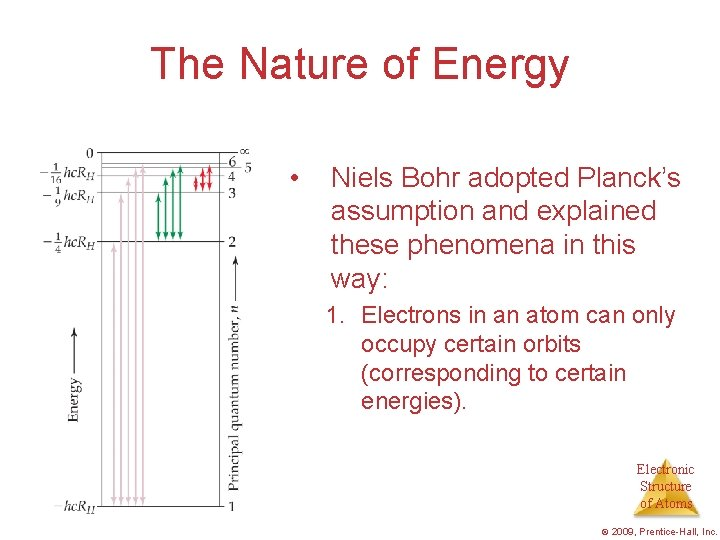The Nature of Energy • Niels Bohr adopted Planck’s assumption and explained these phenomena in this way: 1. Electrons in an atom can only occupy certain orbits (corresponding to certain energies). Electronic Structure of Atoms © 2009, Prentice-Hall, Inc.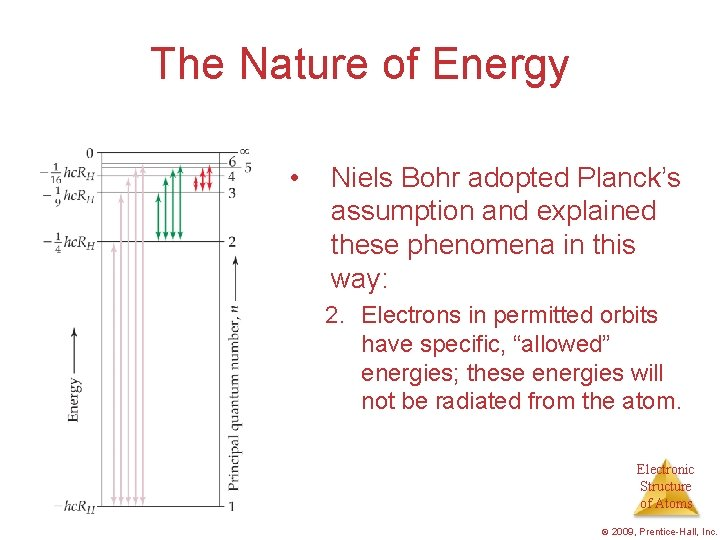The Nature of Energy • Niels Bohr adopted Planck’s assumption and explained these phenomena in this way: 2. Electrons in permitted orbits have specific, “allowed” energies; these energies will not be radiated from the atom. Electronic Structure of Atoms © 2009, Prentice-Hall, Inc.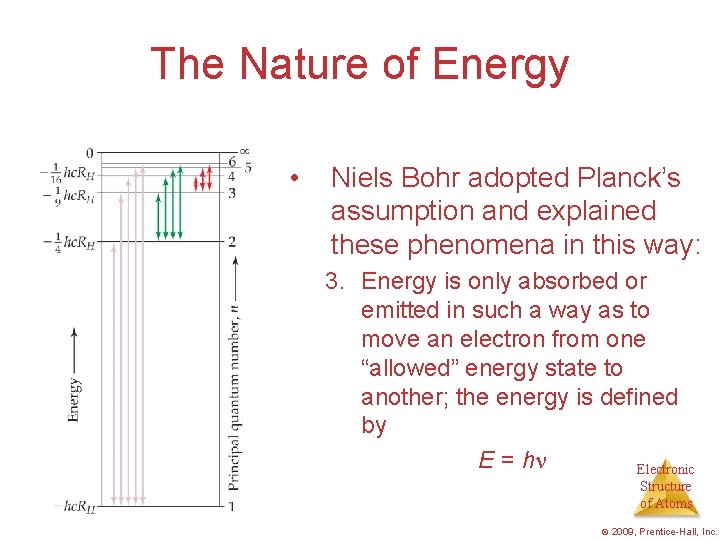The Nature of Energy • Niels Bohr adopted Planck’s assumption and explained these phenomena in this way: 3. Energy is only absorbed or emitted in such a way as to move an electron from one “allowed” energy state to another; the energy is defined by E = h Electronic Structure of Atoms © 2009, Prentice-Hall, Inc.The Nature of Energy The energy absorbed or emitted from the process of electron promotion or demotion can be calculated by the equation: E = −RH ( 1 1 - 2 ni nf 2 ) where RH is the Rydberg constant, 2. 18 10− 18 J, and ni and nf are the initial and final energy levels of the electron. Electronic Structure of Atoms © 2009, Prentice-Hall, Inc.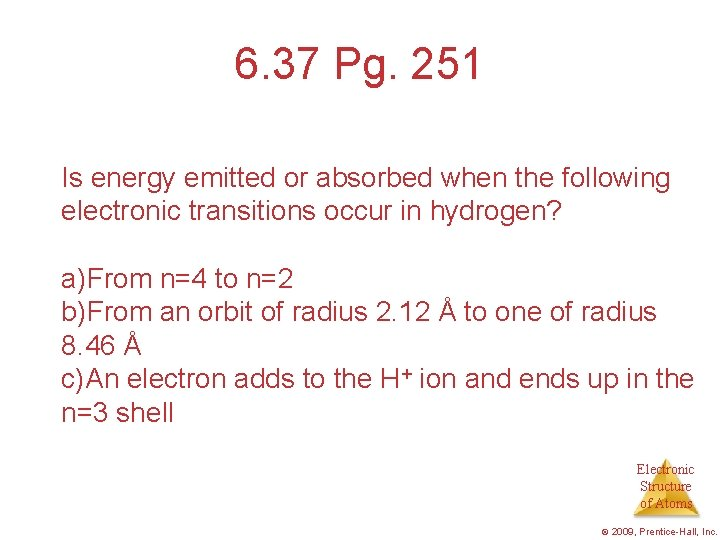6. 37 Pg. 251 Is energy emitted or absorbed when the following electronic transitions occur in hydrogen? a)From n=4 to n=2 b)From an orbit of radius 2. 12 Å to one of radius 8. 46 Å c)An electron adds to the H+ ion and ends up in the n=3 shell Electronic Structure of Atoms © 2009, Prentice-Hall, Inc.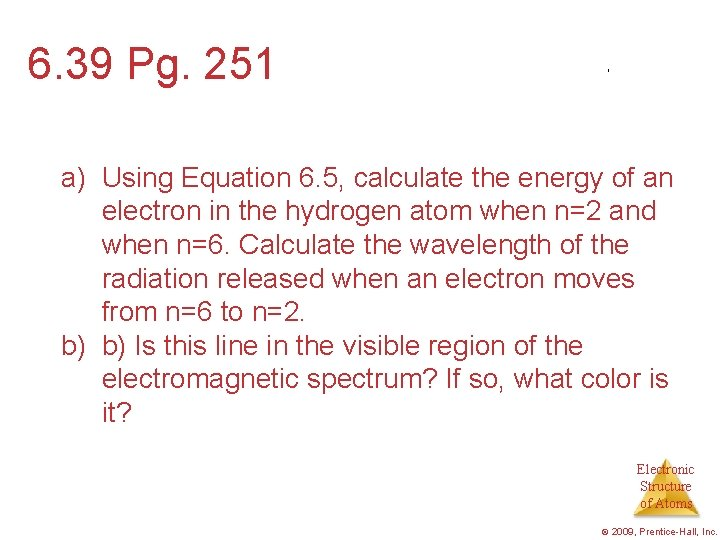6. 39 Pg. 251 a) Using Equation 6. 5, calculate the energy of an electron in the hydrogen atom when n=2 and when n=6. Calculate the wavelength of the radiation released when an electron moves from n=6 to n=2. b) b) Is this line in the visible region of the electromagnetic spectrum? If so, what color is it? Electronic Structure of Atoms © 2009, Prentice-Hall, Inc.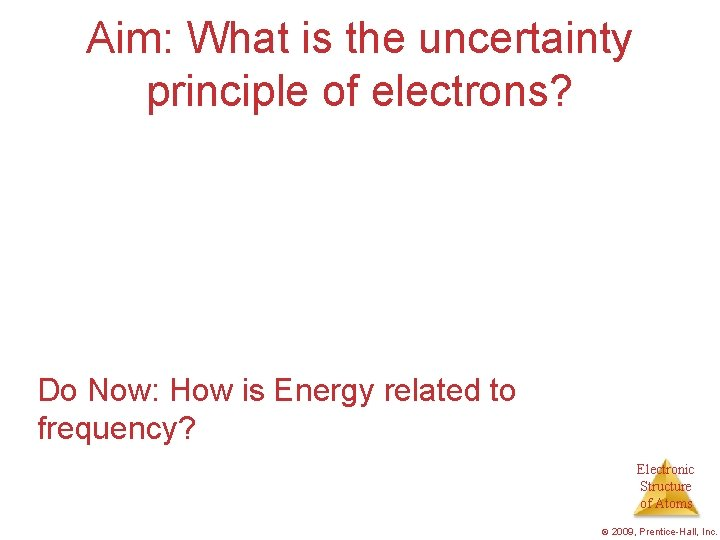Aim: What is the uncertainty principle of electrons? Do Now: How is Energy related to frequency? Electronic Structure of Atoms © 2009, Prentice-Hall, Inc.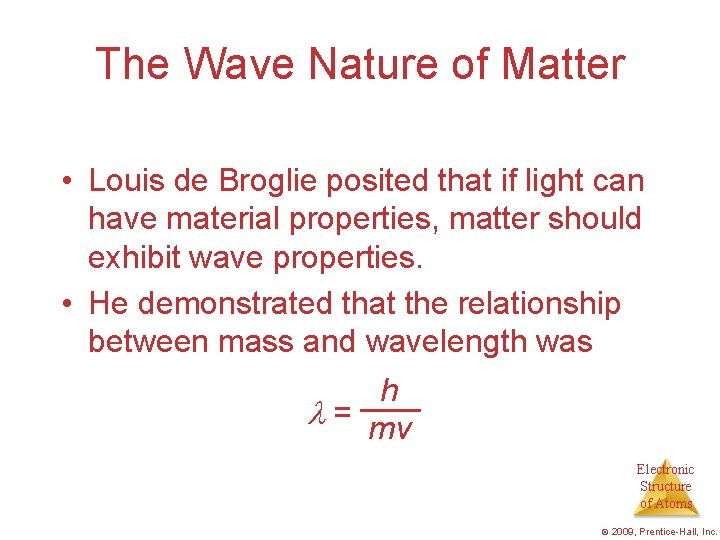The Wave Nature of Matter • Louis de Broglie posited that if light can have material properties, matter should exhibit wave properties. • He demonstrated that the relationship between mass and wavelength was h = mv Electronic Structure of Atoms © 2009, Prentice-Hall, Inc.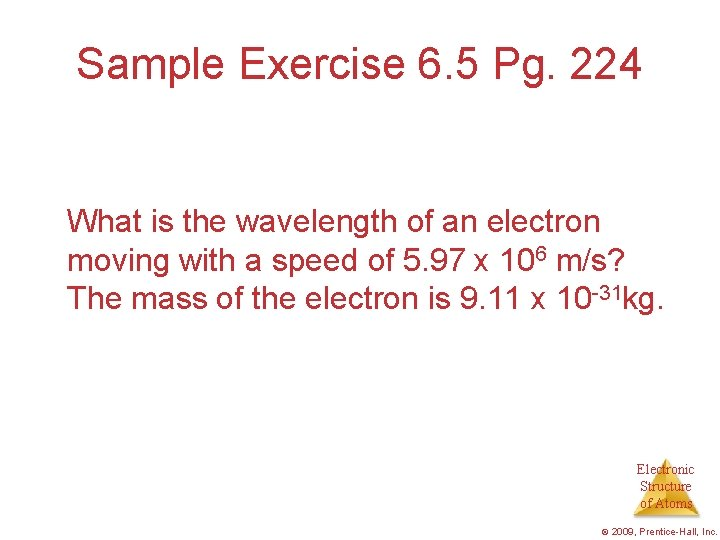Sample Exercise 6. 5 Pg. 224 What is the wavelength of an electron moving with a speed of 5. 97 x 106 m/s? The mass of the electron is 9. 11 x 10 -31 kg. Electronic Structure of Atoms © 2009, Prentice-Hall, Inc.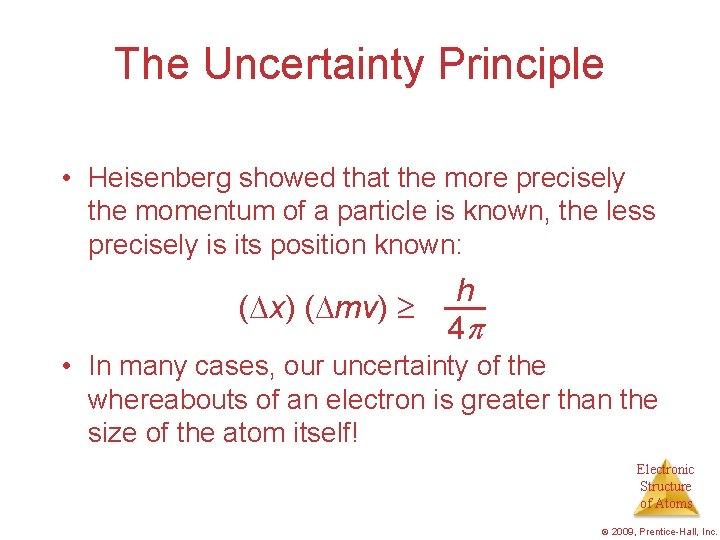The Uncertainty Principle • Heisenberg showed that the more precisely the momentum of a particle is known, the less precisely is its position known: ( x) ( mv) h 4 • In many cases, our uncertainty of the whereabouts of an electron is greater than the size of the atom itself! Electronic Structure of Atoms © 2009, Prentice-Hall, Inc.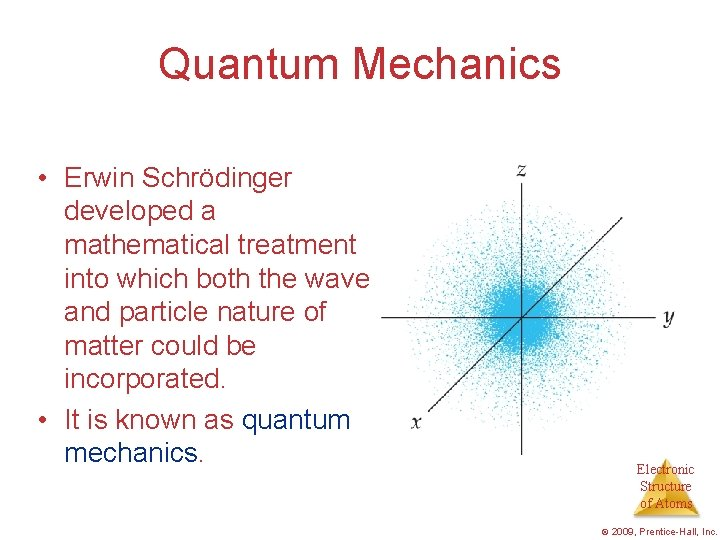Quantum Mechanics • Erwin Schrödinger developed a mathematical treatment into which both the wave and particle nature of matter could be incorporated. • It is known as quantum mechanics. Electronic Structure of Atoms © 2009, Prentice-Hall, Inc.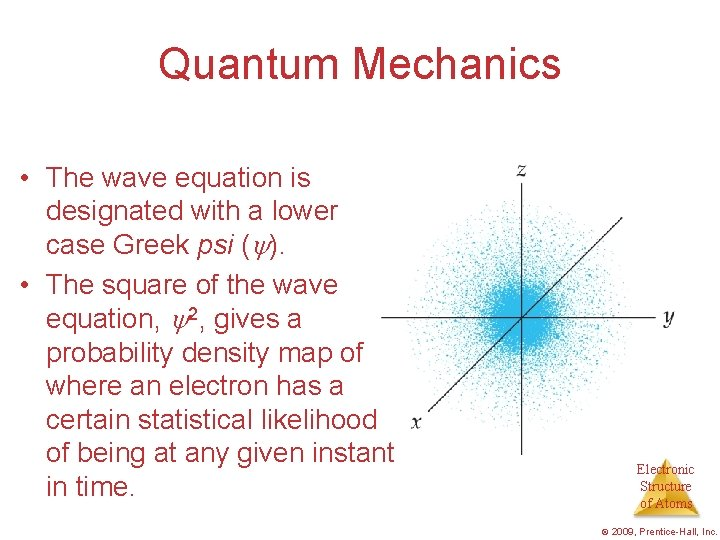Quantum Mechanics • The wave equation is designated with a lower case Greek psi ( ). • The square of the wave equation, 2, gives a probability density map of where an electron has a certain statistical likelihood of being at any given instant in time. Electronic Structure of Atoms © 2009, Prentice-Hall, Inc.Quantum Numbers • Solving the wave equation gives a set of wave functions, or orbitals, and their corresponding energies. • Each orbital describes a spatial distribution of electron density. • An orbital is described by a set of three quantum numbers. Electronic Structure of Atoms © 2009, Prentice-Hall, Inc.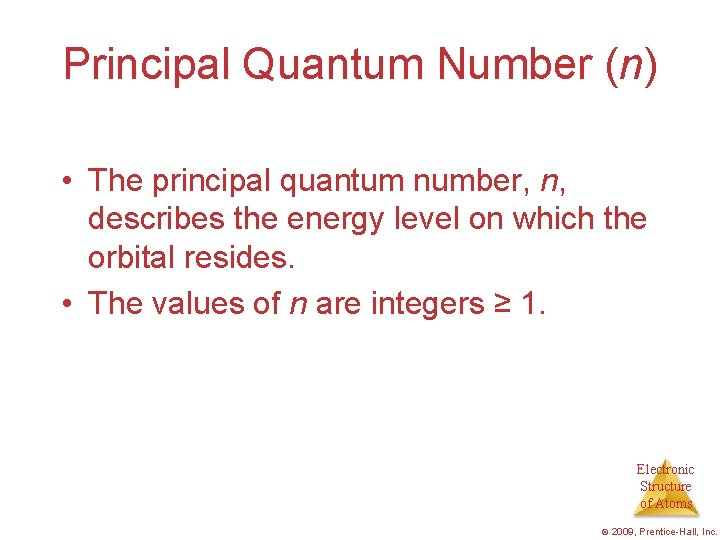Principal Quantum Number (n) • The principal quantum number, n, describes the energy level on which the orbital resides. • The values of n are integers ≥ 1. Electronic Structure of Atoms © 2009, Prentice-Hall, Inc.Angular Momentum Quantum Number (l) • This quantum number defines the shape of the orbital. • Allowed values of l are integers ranging from 0 to n − 1. • We use letter designations to communicate the different values of l and, therefore, the shapes and types of Electronic orbitals. Structure of Atoms © 2009, Prentice-Hall, Inc.Angular Momentum Quantum Number (l) Value of l 0 1 2 3 Type of orbital s p d f Electronic Structure of Atoms © 2009, Prentice-Hall, Inc.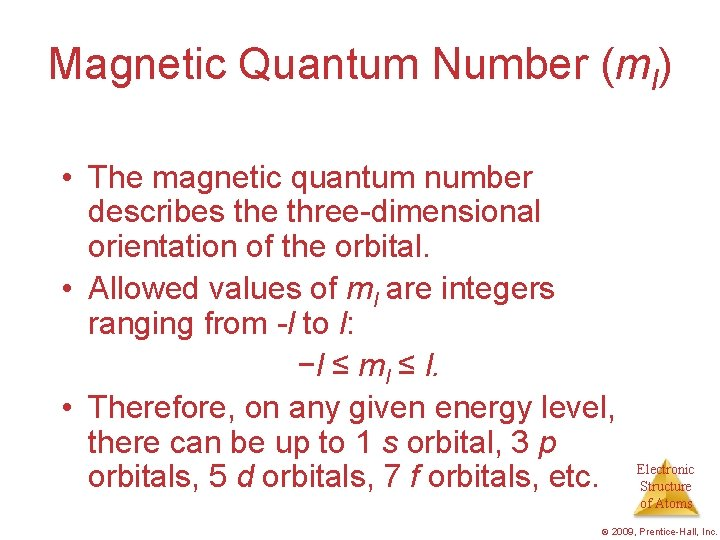Magnetic Quantum Number (ml) • The magnetic quantum number describes the three-dimensional orientation of the orbital. • Allowed values of ml are integers ranging from -l to l: −l ≤ ml ≤ l. • Therefore, on any given energy level, there can be up to 1 s orbital, 3 p orbitals, 5 d orbitals, 7 f orbitals, etc. Electronic Structure of Atoms © 2009, Prentice-Hall, Inc.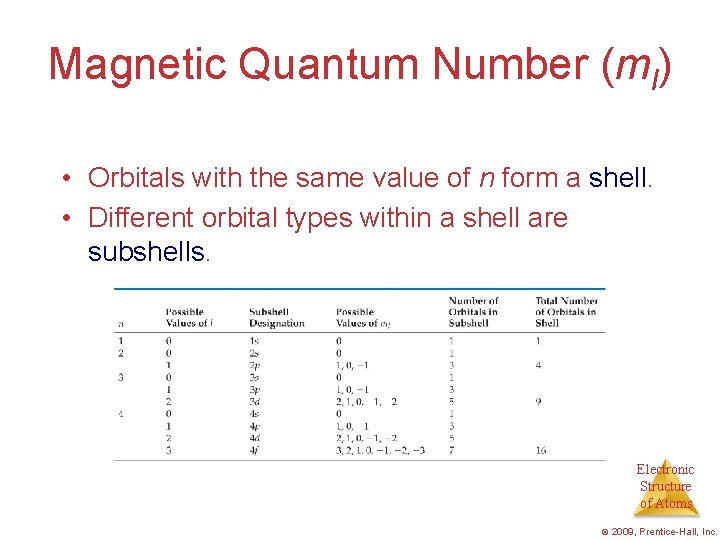Magnetic Quantum Number (ml) • Orbitals with the same value of n form a shell. • Different orbital types within a shell are subshells. Electronic Structure of Atoms © 2009, Prentice-Hall, Inc.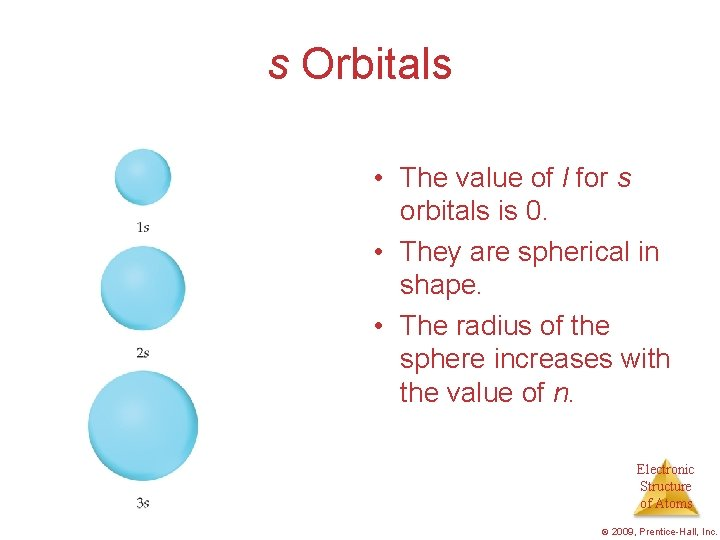s Orbitals • The value of l for s orbitals is 0. • They are spherical in shape. • The radius of the sphere increases with the value of n. Electronic Structure of Atoms © 2009, Prentice-Hall, Inc.s Orbitals Observing a graph of probabilities of finding an electron versus distance from the nucleus, we see that s orbitals possess n− 1 nodes, or regions where there is 0 probability of finding an electron. Electronic Structure of Atoms © 2009, Prentice-Hall, Inc.p Orbitals • The value of l for p orbitals is 1. • They have two lobes with a node between them. Electronic Structure of Atoms © 2009, Prentice-Hall, Inc.d Orbitals • The value of l for a d orbital is 2. • Four of the five d orbitals have 4 lobes; the other resembles a p orbital with a doughnut around the center. Electronic Structure of Atoms © 2009, Prentice-Hall, Inc.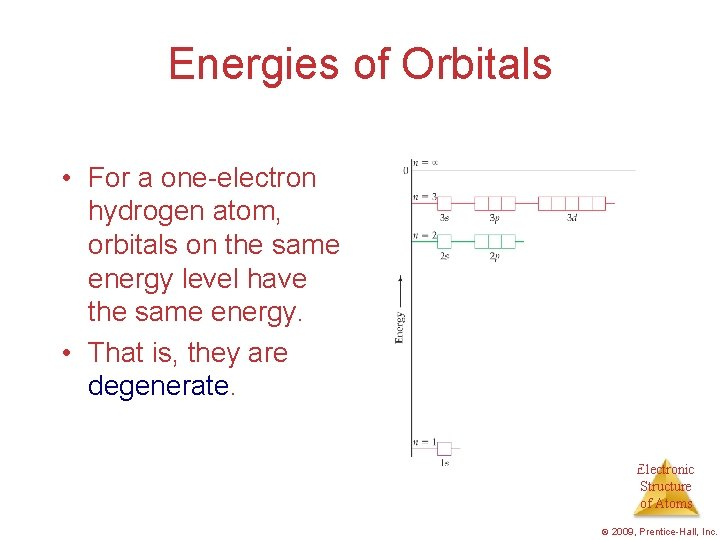Energies of Orbitals • For a one-electron hydrogen atom, orbitals on the same energy level have the same energy. • That is, they are degenerate. Electronic Structure of Atoms © 2009, Prentice-Hall, Inc.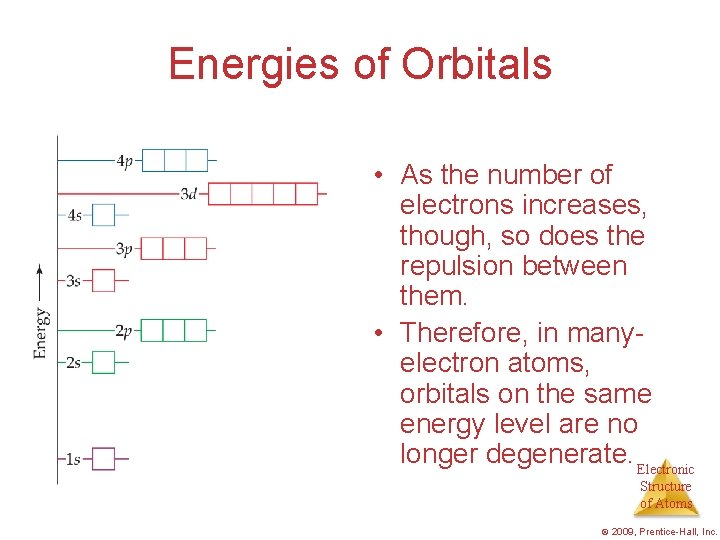Energies of Orbitals • As the number of electrons increases, though, so does the repulsion between them. • Therefore, in manyelectron atoms, orbitals on the same energy level are no longer degenerate. Electronic Structure of Atoms © 2009, Prentice-Hall, Inc.Spin Quantum Number, ms • In the 1920 s, it was discovered that two electrons in the same orbital do not have exactly the same energy. • The “spin” of an electron describes its magnetic field, which affects its energy. Electronic Structure of Atoms © 2009, Prentice-Hall, Inc.Spin Quantum Number, ms • This led to a fourth quantum number, the spin quantum number, ms. • The spin quantum number has only 2 allowed values: +1/2 and − 1/2. Electronic Structure of Atoms © 2009, Prentice-Hall, Inc.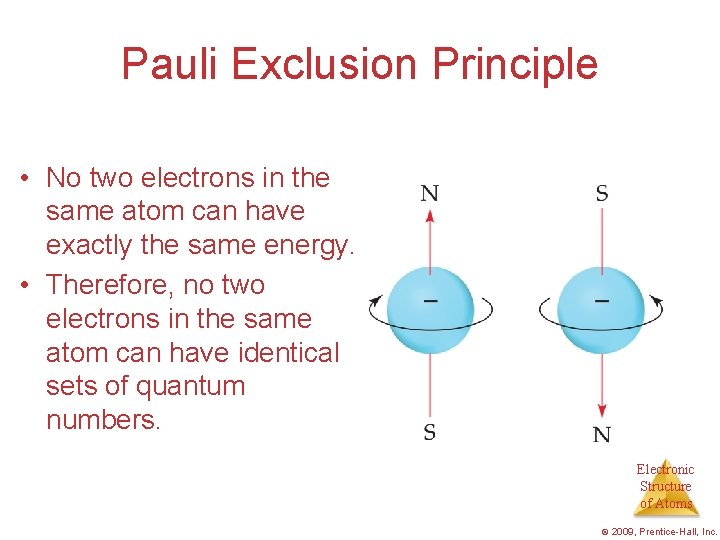Pauli Exclusion Principle • No two electrons in the same atom can have exactly the same energy. • Therefore, no two electrons in the same atom can have identical sets of quantum numbers. Electronic Structure of Atoms © 2009, Prentice-Hall, Inc.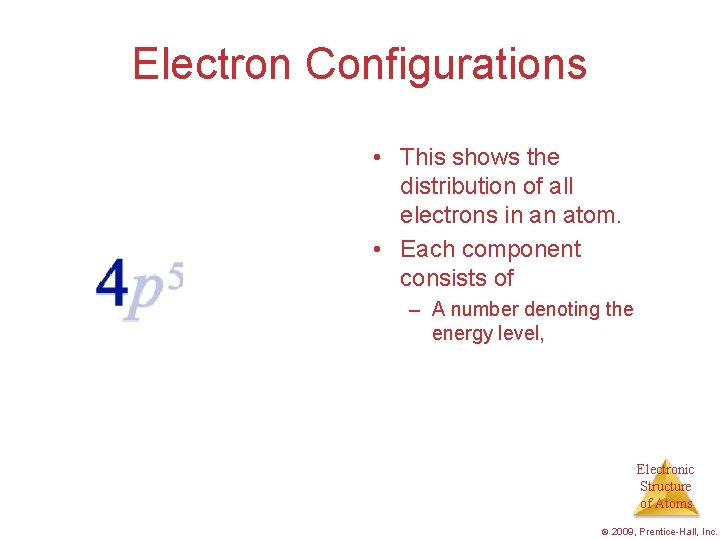Electron Configurations • This shows the distribution of all electrons in an atom. • Each component consists of – A number denoting the energy level, Electronic Structure of Atoms © 2009, Prentice-Hall, Inc.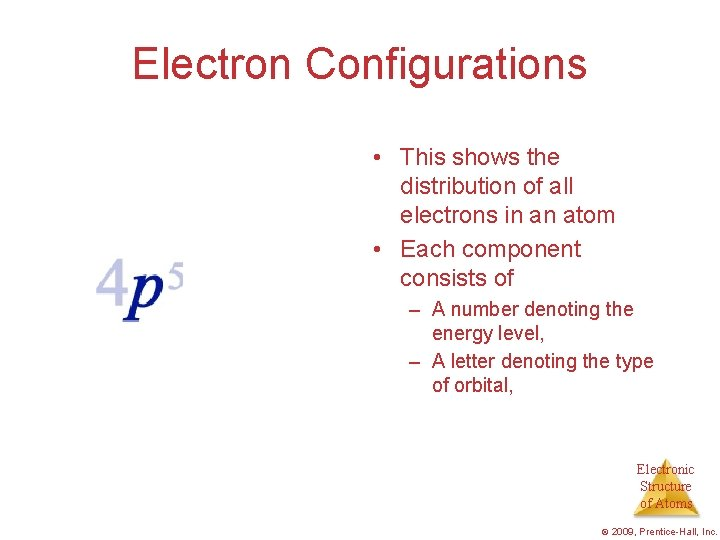Electron Configurations • This shows the distribution of all electrons in an atom • Each component consists of – A number denoting the energy level, – A letter denoting the type of orbital, Electronic Structure of Atoms © 2009, Prentice-Hall, Inc.Electron Configurations • This shows the distribution of all electrons in an atom. • Each component consists of – A number denoting the energy level, – A letter denoting the type of orbital, – A superscript denoting the number of electrons in those orbitals. Electronic Structure of Atoms © 2009, Prentice-Hall, Inc.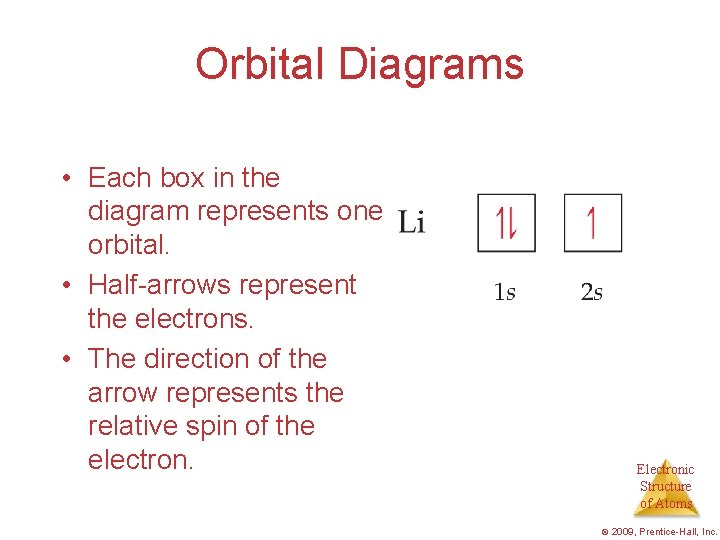Orbital Diagrams • Each box in the diagram represents one orbital. • Half-arrows represent the electrons. • The direction of the arrow represents the relative spin of the electron. Electronic Structure of Atoms © 2009, Prentice-Hall, Inc.Hund’s Rule “For degenerate orbitals, the lowest energy is attained when the number of electrons with the same spin is maximized. ” Electronic Structure of Atoms © 2009, Prentice-Hall, Inc.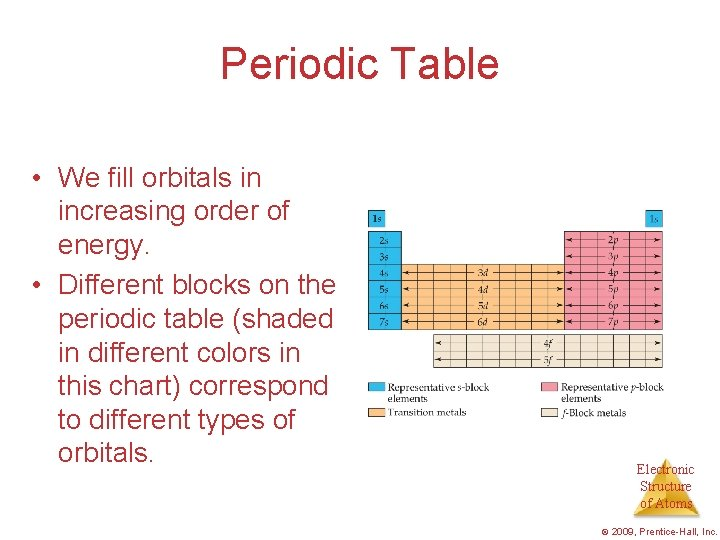Periodic Table • We fill orbitals in increasing order of energy. • Different blocks on the periodic table (shaded in different colors in this chart) correspond to different types of orbitals. Electronic Structure of Atoms © 2009, Prentice-Hall, Inc.Some Anomalies Some irregularities occur when there are enough electrons to halffill s and d orbitals on a given row. Electronic Structure of Atoms © 2009, Prentice-Hall, Inc.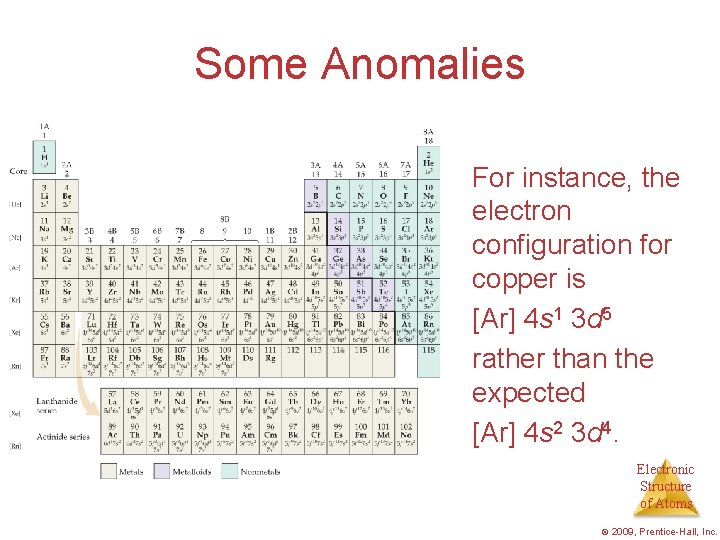Some Anomalies For instance, the electron configuration for copper is [Ar] 4 s 1 3 d 5 rather than the expected [Ar] 4 s 2 3 d 4. Electronic Structure of Atoms © 2009, Prentice-Hall, Inc.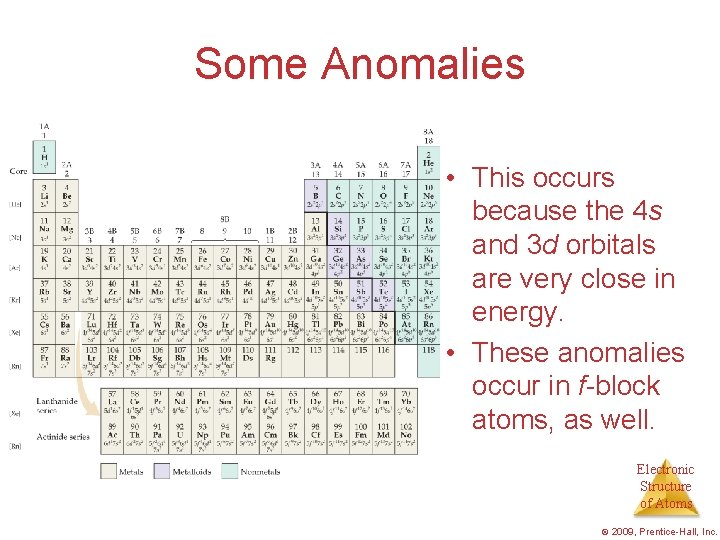Some Anomalies • This occurs because the 4 s and 3 d orbitals are very close in energy. • These anomalies occur in f-block atoms, as well. Electronic Structure of Atoms © 2009, Prentice-Hall, Inc.